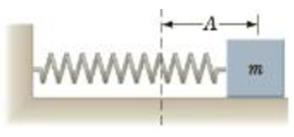Chapter 13, Problem 1P

Chapter
Section
Textbook Problem

A block, of mass m = 0.60 kg attached to a spring with force; constant 130 N/m is free to move on a frictionless, horizontal surface as in Figure P13.1. The block is released from rest alter the spring is stretched a distance A = 0.13 m. At that instant, find (a) the force on the block and (b) its acceleration.Figure P13.1

a)

To determine
The force on the block.

Explanation

Given info: The mass of the block is 0.60 Kg. The force constant of the spring is 130Nm-1 . The block is stretched to 0.13 m.

Explanation:

In simple harmonic motion the net force applied along the direction of motion follows Hook’s law:

F=kx

Here,

F is the restoring force on the block.

k is force constant of the spring.

x is the displacement of the spring.

Consider the right side as the positive direction. Substitute 130Nm-1 for k and 0

b)

To determine
The acceleration of the block.

Still sussing out bartleby?

Check out a sample textbook solution.

See a sample solution

The Solution to Your Study Problems

Bartleby provides explanations to thousands of textbook problems written by our experts, many with advanced degrees!

Get Started

Show that 1 cubic meter contains 1000 L.

An Introduction to Physical Science

Compare the lock-and-key theory with the induced-fit theory.

Chemistry for Today: General, Organic, and Biochemistry

What are the features of the continental margins?

Oceanography: An Invitation To Marine Science, Loose-leaf Versin